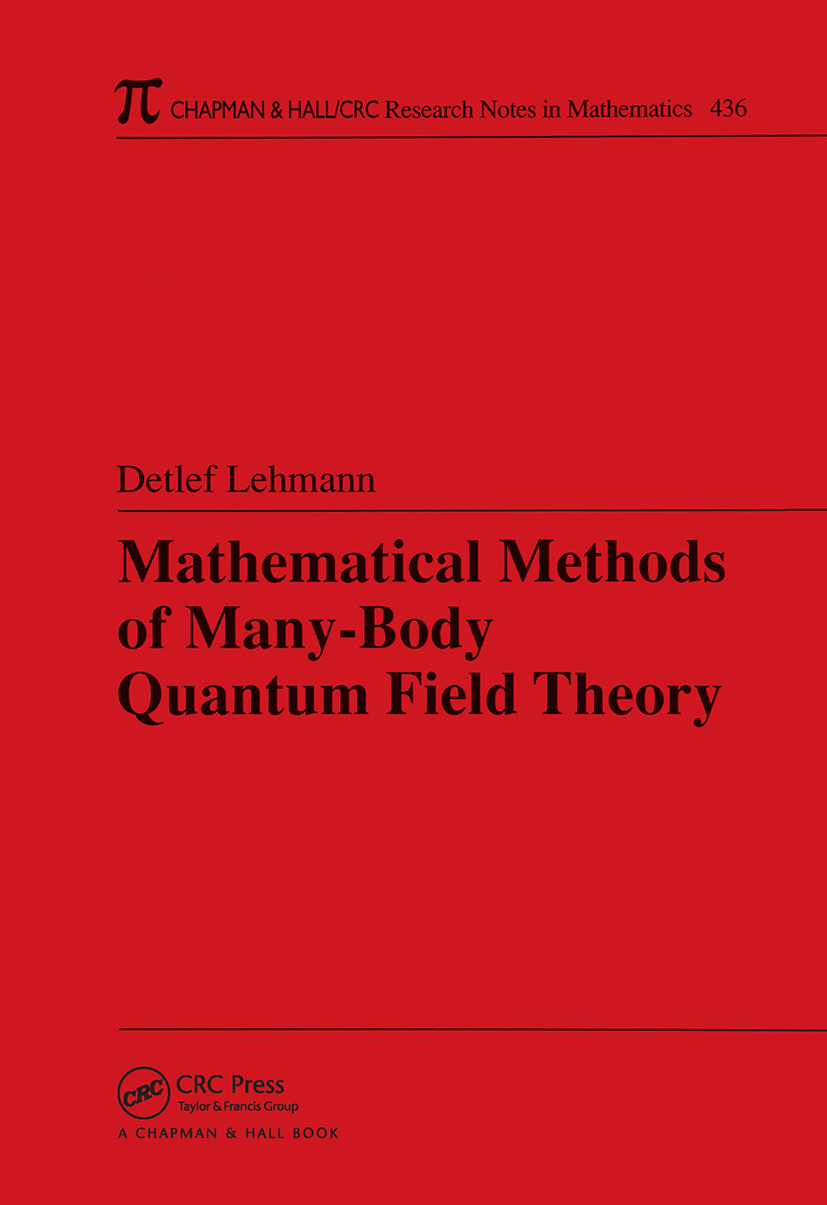Mathematical Methods of Many-Body Quantum Field Theory

1st Edition

Chapman and Hall/CRC

264 pages | 22 B/W Illus.

Purchasing Options:\$ = USD
Paperback: 9780367393908
pub: 2019-09-05
SAVE ~\$14.99
\$74.95
\$59.96
x
Hardback: 9781584884903
pub: 2004-08-30
SAVE ~\$34.00
\$170.00
\$136.00
x
eBook (VitalSource) : 9780429178207
pub: 2004-08-30
from \$28.98

FREE Standard Shipping!

Description

Mathematical Methods of Many-Body Quantum Field Theory offers a comprehensive, mathematically rigorous treatment of many-body physics. It develops the mathematical tools for describing quantum many-body systems and applies them to the many-electron system. These tools include the formalism of second quantization, field theoretical perturbation theory, functional integral methods, bosonic and fermionic, and estimation and summation techniques for Feynman diagrams. Among the physical effects discussed in this context are BCS superconductivity, s-wave and higher l-wave, and the fractional quantum Hall effect. While the presentation is mathematically rigorous, the author does not focus solely on precise definitions and proofs, but also shows how to actually perform the computations.

Presenting many recent advances and clarifying difficult concepts, this book provides the background, results, and detail needed to further explore the issue of when the standard approximation schemes in this field actually work and when they break down. At the same time, its clear explanations and methodical, step-by-step calculations shed welcome light on the established physics literature.

Reviews

"The book is clearly written, and all computations are performed in full detail."

Mathematical Reviews

"The presentation is mathematically rigorous, where possible. The author’s aim was to create a book containing enough motivation and enough mathematical details for those interested in this advanced and important field of contemporary mathematical physics."

—European Mathematical Society

INTRODUCTION

SECOND QUANTIZATION

Coordinate and Momentum Space

The Many-Electron System

Annihilation and Creation Operators

PERTURBATION THEORY

The Perturbation Series for e(H0+lV)

The Perturbation Series for the Partition Function

The Perturbation Series for the Correlation Functions

GAUSSIAN INTEGRATION AND GRASSMANN INTEGRALS

Why Grassmann Integration? A Motivating Example

Grassmann Integral Representations

Ordinary Gaussian Integrals

Theory of Grassmann Integration

BOSONIC FUNCTIONAL INTEGRAL REPRESENTATION

The Hubbard Stratonovich Transformation

The Effective Potential

BCS THEORY AND SPONTANEOUS SYMMETRY BREAKING

The Quadratic Mean Field Model

The Quartic BCS Model

BCS with Higher l-Wave Interaction

THE MANY-ELECTRON SYSTEM IN A MAGNETIC FIELD

Solution of the Single Body Problem

Diagonalization of the Fractional Quantum Hall Hamiltonian

FEYNMAN DIAGRAMS

The Typical Behavior of Field Theoretical Perturbation Series

Connected Diagrams and the Linked Cluster Theorem

Estimates on Feynman Diagrams

RENORMALIZATION GROUP METHODS

Integrating Out Scales

A Single Scale Bound on the Sum of all Diagrams

A Multiscale Bound on the Sum of Convergent Diagrams

Elimination of Divergent Diagrams

The Feldman-Knorrer-Trubowitz Fermi Liquid Construction

RESUMMATION OF PERTURBATION SERIES

Starting Point and Typical Examples

Computing Inverse Matrix Elements

The Averaged Greens Function of the Anderson Model

The Many-Electron System with Attractive Delta-Interaction

Application to Bosonic Models

General Structure of the Integral Equations

THE 'MANY-ELECTRON MILLENNIUM PROBLEMS'

REFERENCES`

Lehmann, Detlef